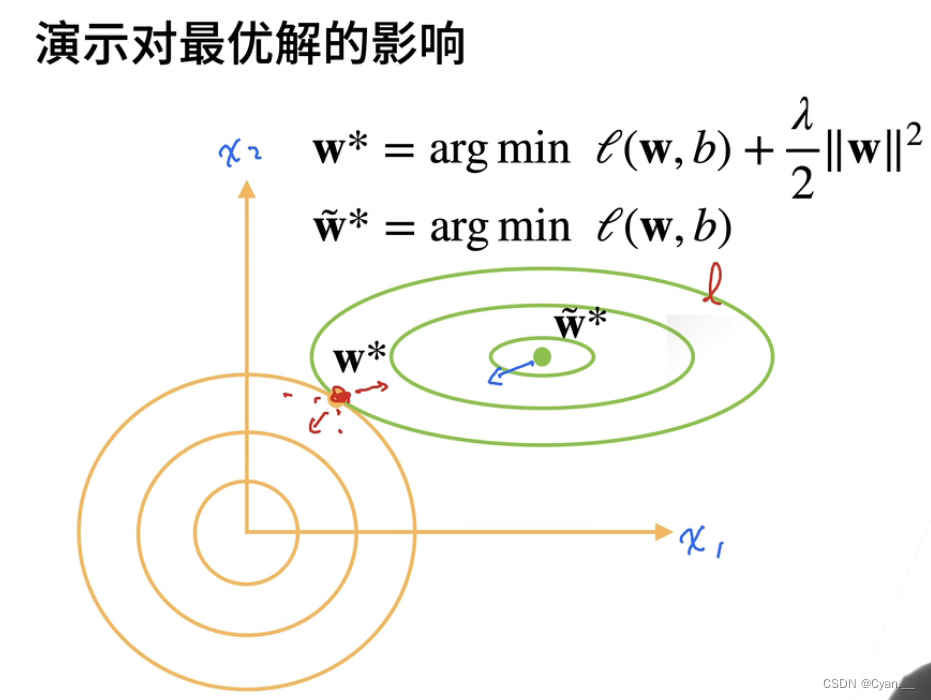## 【机器学习】036_权重衰退

news/2023/12/1 1:22:31

### 一、范数

#### · 定义：向量的范数表示一个向量有多大（分量的大小）

L1范数：

· 即向量元素绝对值之和，用符号 ‖ v ‖ 1 表示。

· 公式：L2范数：

· 即向量的模，向量各元素绝对值的平方之和再开根号，用符号 ‖ v ‖ 2 表示。

· 公式：Lp范数：

· 即向量范数的一般形式，各元素绝对值的p次幂之和再开p次根号，用符号 ‖ v ‖ p 表示。

· 公式：### 二、权重衰减（L2正则化）

#### 模型（函数）复杂度的度量：

· 一般通过线性函数中的权重向量的某个范数（如）来度量其复杂度

· 通过限制参数值的选择范围来控制模型容量

#### 衰减方法：· 其中，是模型原本的损失函数，是新添加的惩罚项。

· 正则化常数用来描绘这种权衡，其为一个非负超参数。

·的值越大，表示对的约束较大；反之的值越小，表示对的约束较小。

※为何选用平方范数而不是标准范数：

· 便于计算。平方范数可以去掉平方根使得导数更容易计算，利于反向传播过程。

· 使用L2范数是因为它会对权重向量的大分量施加巨大的惩罚，使各权重均匀分布。

· L1范数惩罚会导致权重集中在某一小部分特征上，其它权重被清除为0（特征选择）。现在在等高线图上，梯度下降最终收敛的位置不再是某一个项所造成的最低点，因为在这时，可能误差项达到最小了，但是惩罚项很大，使得惩罚项拉着损失函数再向另一个方向移动。

只有当达到了两个项共同作用下的一个平衡点时，损失函数才具有最小值，这个时候的模型往往复杂度也降低了，虽然有可能造成训练损失增大，但是测试损失会减小。

### 三、代码实现权重衰减

#### 从零实现代码如下：

import matplotlib
import torch
from torch import nn
from d2l import torch as d2l# 训练数据集、测试数据集、输入值、训练批次
n_train, n_test, num_inputs, batch_size = 20, 100, 200, 5
# 初始化w和b的真实值
true_w, true_b = torch.ones((num_inputs, 1)) * 0.01, 0.05
# 拿到训练数据
train_data = d2l.synthetic_data(true_w, true_b, n_train)
test_data = d2l.synthetic_data(true_w, true_b, n_test)
test_iter = d2l.load_array(test_data, batch_size, is_train=False)# 初始化模型参数w和b
def init_params():w = torch.normal(0, 1, size=(num_inputs, 1), requires_grad=True)b = torch.zeros(1, requires_grad=True)return [w, b]
# 定义L2范数惩罚项
# 实现训练代码，读入参数为兰姆达（正则化参数）
def train(lambd):w, b = init_params()net, loss = lambda X: d2l.linreg(X, w, b), d2l.squared_lossnum_epochs, lr = 100, 0.003animator = d2l.Animator(xlabel='epochs', ylabel='loss', yscale='log',xlim=[5, num_epochs], legend=['train', 'test'])for epoch in range(num_epochs):for X, y in train_iter:# 增加了L2范数惩罚项，# 广播机制使l2_penalty(w)成为一个长度为batch_size的向量l = loss(net(X), y) + lambd * l2_penalty(w)l.sum().backward()d2l.sgd([w, b], lr, batch_size)if (epoch + 1) % 5 == 0:animator.add(epoch + 1, (d2l.evaluate_loss(net, train_iter, loss),d2l.evaluate_loss(net, test_iter, loss)))print('w的L2范数是：', torch.norm(w).item())
# 使用权重进行训练
train(lambd=3)


#### 简洁实现代码如下：

import torch
from torch import nn
from d2l import torch as d2l# 训练数据集、测试数据集、输入值、训练批次
n_train, n_test, num_inputs, batch_size = 20, 100, 200, 5
# 初始化w和b的真实值
true_w, true_b = torch.ones((num_inputs, 1)) * 0.01, 0.05
# 拿到训练数据
train_data = d2l.synthetic_data(true_w, true_b, n_train)
test_data = d2l.synthetic_data(true_w, true_b, n_test)
test_iter = d2l.load_array(test_data, batch_size, is_train=False)def train_concise(wd):net = nn.Sequential(nn.Linear(num_inputs, 1))for param in net.parameters():param.data.normal_()loss = nn.MSELoss(reduction='none')num_epochs, lr = 100, 0.003# 偏置参数没有衰减trainer = torch.optim.SGD([{"params":net.weight,'weight_decay': wd},{"params":net.bias}], lr=lr)animator = d2l.Animator(xlabel='epochs', ylabel='loss', yscale='log',xlim=[5, num_epochs], legend=['train', 'test'])for epoch in range(num_epochs):for X, y in train_iter:trainer.zero_grad()l = loss(net(X), y)l.mean().backward()trainer.step()if (epoch + 1) % 5 == 0:animator.add(epoch + 1,(d2l.evaluate_loss(net, train_iter, loss),d2l.evaluate_loss(net, test_iter, loss)))print('w的L2范数：', net.weight.norm().item())train_concise(3)

### 【Linux】 find命令使用

find find命令是一种通过条件匹配在指定目录下查找对应文件或者目录的工具。匹配的条件可以是文件名称、类型、大小、权限属性、时间戳等。find命令还可以配合相关命令对匹配到的文件作出后续处理。 语法 find [路径...] [表达式] [path...]为需要查找文件所指定的路径。如果…

### Spring Boot和Spring MVC的区别

1 Spring MVC 是Spring的一个模块&#xff0c;是一个web框架。分为Model&#xff0c;View&#xff0c;Controller&#xff08;模型层、视图层、控制层&#xff09;。 2 Spring Boot Spring Boot 自动配置&#xff0c;降低了项目搭建的复杂度。Spring框架需要大量的配置&…

### Kubernetes Gateway API 攻略：解锁集群流量服务新维度！

Kubernetes Gateway API 刚刚 GA&#xff0c;旨在改进将集群服务暴露给外部的过程。这其中包括一套更标准、更强大的 API资源&#xff0c;用于管理已暴露的服务。在这篇文章中&#xff0c;我将介绍 Gateway API 资源&#xff0c;并以 Istio 为例来展示这些资源是如何关联的。通…